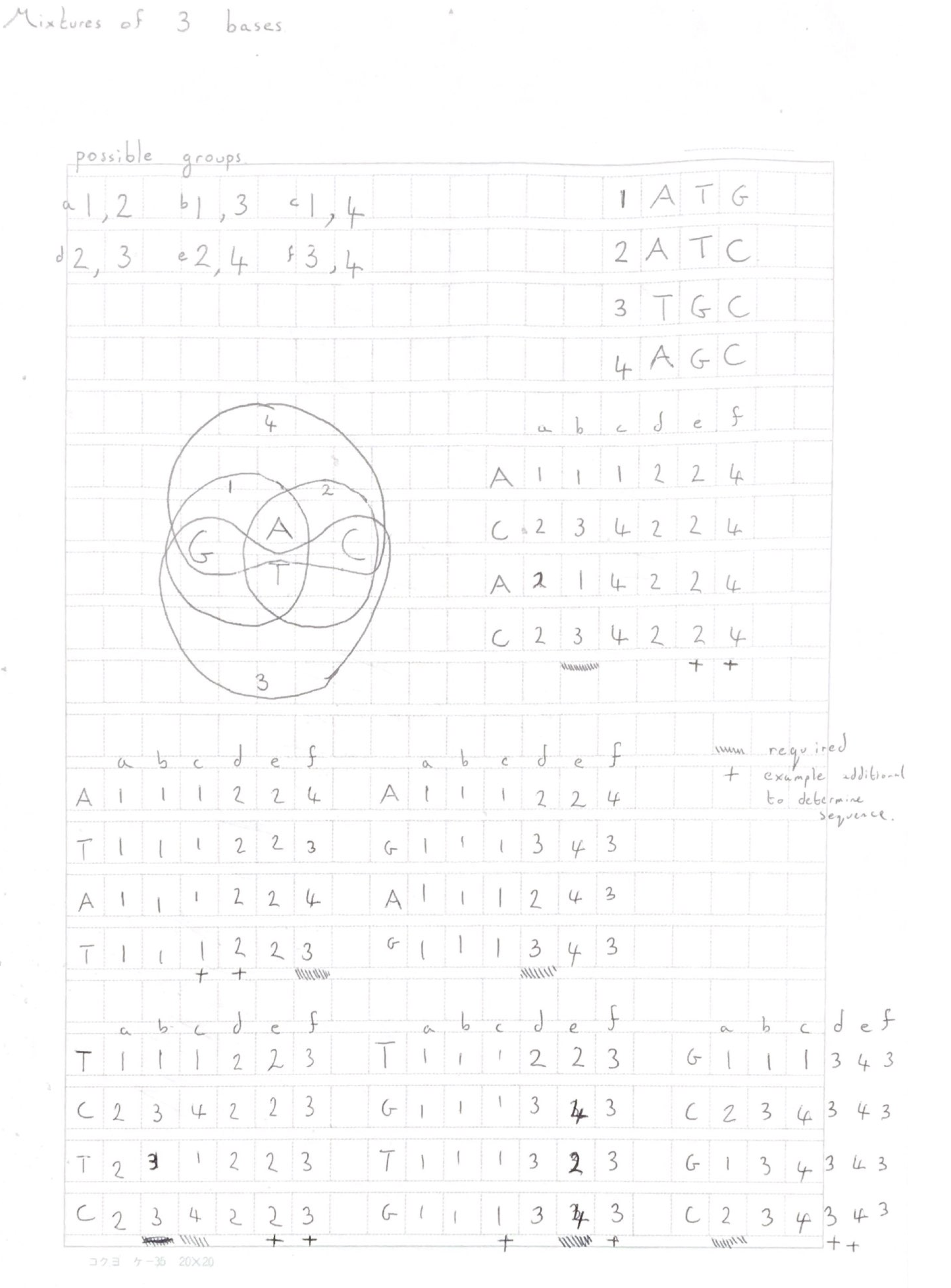## Sequencing with Mixtures of Three BasesA previous post discussed Cygnus’ approach to sequencing, using mixtures of bases and multiple reads of the same template. Centrillion also have a patent that appears to cover a related approach.

The Cygnus approach, as described in their paper uses mixtures of 2 bases. I thought it might be interesting to work through corrections using mixtures of 3 bases. It’s possible this is covered somewhere in their supplementary info, or huge 200+ page patent. I’ve not checked and this is just for fun.

There are 4 possible sets of 3 different base types: ATG, ATC, TGC and AGC. The difference between each of these sets is clearly a single base (3 bases out of ATGC in the set, and 1 left out).

To recap on the previous post, a template is exposed to alternating sets (mixtures) of bases, and we measure incorporation intensity and learn how many bases incorporate (as in the same for a normal single channel unterminated sequencing chemistry). In order to process the entire strand the sets we alternate between must contain all base types. For the sets of 3 base types this is no problem, any pair of sets will contain all four base types and differ by only a single base type.

There are 6 possible pairings:

a ATG,ATC

b ATG,TGC

c ATG,AGC

d ATC,TGC

e ATC,AGC

f TGC,AGC

We could vary the order of the pairs. But we don’t really need to. Working through all possible 2bp repeats  it’s clear that we can accurate resolve all sequences using 3 out of the 6 alternating pairs.

In all cases, one pairing supplies the base transition information. For example for the repeat ATATAT this is group f above. This is the only pairing that blocks incorporation between A and T transitions. Each pairing blocks on transitions between one of the six possible transition types (G<->C A<->T A<->G A<->C T<->G T<->C). To accurately resolve all sequences, all pairings are therefore required. In the example 2bp repeats, one pairing provides the “transition” information and 2 other pairings are required to resolve the sequence to one of the four bases.

You therefore need to sequence each template six times. However, at any given base information from only 3 of the “mixture sequences” is required to resolve the strand. The other 3 sequences provide redundant information for error correction. This information could be used in a number of ways (either masking likely errored bases, taking a majority vote, or using this information in a more complex error correction model).

How much sequencing does this require as compared to standard single base sequencing?

Well, there will always be degenerate sequences, both in this scheme and the Cygnus approach. These sequences will require very slightly more sequencing than using a normal single base incorporation system.

However we can simulate the number of cycles required (a cycle being the incorporation of a single base type, or a single mixture type). I quickly threw some code together to do this . Assuming this hastily thrown together code is correct the single base incorporation scheme requires 1.481 cycles per base (or ~2.7 bases incorporated per set of 4 bases). The mix of 3 scheme described above requires 1.4905 cycles per base.

So, if you just go by this, there’s very little overhead.

One downside of the base mixture incorporations is that the sequencing system has to cope with longer homopolymers (or rather runs of 1 of 3 different base types). Again this is true of the approach described here, and the Cygnus system. What issues this causes, will depend on the error profile of the underlying technology.

While I’ve discussed mixtures of 3 bases here, it might also be interesting to look at combinations of mixtures of 2 and 3 bases. For example you might have set pairs of ATG, and ATC. Then a set of CA and GT to resolve the ambiguity (this could be extended to create a complete sequencing system).

Maybe that’s another fun project for another time.

## Notes```#include <iostream>
#include <vector>
#include <math.h>
#include <stdlib.h>

using namespace std;

// Multiple base incorporations
string s1 = "ATG";
string s2 = "ATC";
string s3 = "TGC";
string s4 = "AGC";

int mix_incorp(string temp,vector<string> pair) {

int p=0;
int cycles=0;
for(int n=0;n<temp.size();) {

for(;;) {
}

cycles++;
if(p==0) p=1; else p=0;
}

return cycles;

}

int main() {

string temp;

// generate random sequence
for(int n=0;n<10000;n++) {
int r = rand()%4;
if(r == 0) temp += "A";
if(r == 1) temp += "T";
if(r == 2) temp += "G";
if(r == 3) temp += "C";
}

cout << "Sequence: " << temp << endl;

// Single base incorps
string order="ATGC";
int pos=0;
int cycle_count=0;
for(int n=0;n<temp.size();) {

for(;temp[n] == order[pos];) n++;

pos++;
cycle_count++;
if(pos == order.size()) pos=0;
}
cout << "Average cycles per base, single base incorps: " << ((float)cycle_count)/((float)temp.size()) << endl;

// Super ugly code, but functional...
vector<vector<string> > pairs(6);
pairs.push_back(s1);
pairs.push_back(s2);
pairs.push_back(s1);
pairs.push_back(s3);
pairs.push_back(s1);
pairs.push_back(s4);
pairs.push_back(s2);
pairs.push_back(s3);
pairs.push_back(s2);
pairs.push_back(s4);
pairs.push_back(s3);
pairs.push_back(s4);

int total=0;
for(int n=0;n<6;n++) {

int count = mix_incorp(temp,pairs[n]);
total+=count;
}
cout << "Average cycles per base, mixture incorps: " << ((float)total)/((float)temp.size()) << endl;
}
```